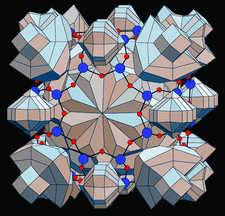issue contentsFOUNDATIONSADVANCES
ISSN: 2053-2733

July 2003 issueCover illustration: Polyhedral representation of voids in the sodium-free sublattice of the crystal structure of NaAlSiO4 zeolite. See Blatov & Shevchenko [Acta Cryst. (2003). A59, 34-44].

research papers

Determination of all misorientations of tetragonal lattices with low multiplicity; connection with Mallard's rule of twinning

Methods to determine the axial ratios of primitive and body-centred tetragonal lattices admitting multiplicities Σ lower than a chosen upper limit are developed and applied to produce lists giving all cases with Σ ≤ 5 and the corresponding twin mirror planes.

Secondary extinction and diffraction behaviors in cylindrical crystals

The power ratio distribution, the integrated power ratio and the secondary-extinction factor for cylindrical crystals with three kinds of mosaic distributions are calculated and are analyzed to reveal their relationship with the diffraction geometry.

Six-beam X-ray section topograph' images and computer-simulated images using the new n-beam dynamical theory based on the Takagi–Taupin equations

A six-beam X-ray section topograph' experiment was performed whose images are in excellent agreement with the images computer-simulated using a theory based on the Takagi–Taupin dynamical theory.

Hexagonal and trigonal sphere packings. I. Invariant and univariant lattice complexes

All homogeneous sphere packings have been derived that refer to the invariant and univariant lattice complexes of the hexagonal crystal family. Numerous examples of corresponding crystal structures are given.

S-SWAT (softer single-wavelength anomalous technique): potential in high-throughput protein crystallography

Two phasing protocols are compared for Xe-apocrustacyanin A1 as a test case. The L1-absorption edge optimized Xe f′′ signal using 2.0 Å wavelength yielded high-quality electron-density maps even with modest redundancy (7) from a single data set.

Improving triplet-phase accuracy by symmetry observations in reference-beam diffraction measurements

Symmetry in reference-beam diffraction experiments leads to a fourfold measurement redundancy of any triplet-phase δ in a complete 360° rotation, with two of the four cases corresponding to measurements of the coupling Friedel's mate that may give rise to enhanced three-beam interference effects.

Charge density and chemical bonding in rutile, TiO2

Accurate charge deformation maps in rutile are obtained by using accurate low-order X-ray structure factors measured by quantitative convergent-beam electron difraction.

Identification of and symmetry computation for crystal nets

Methods of determining the combinatorial symmetry of a net and of determining whether two nets are combinatorially isomorphic are described.

Inflation rule for Gummelt coverings with decorated decagons and its implication for quasi-unit-cell models

Quasi-unit-cell models and Penrose-tile models are equivalent.

short communications

On the symmetry of simple 14- and 15-hedra

The symmetry point-group statistics for all combinatorially non-isomorphic simple 14- (339722) and 15-hedra (2406841 in total) are contributed in the paper for the first time.

Anomalous difference signal in protein crystals

A scattering-angle-dependent formula has been developed and used to estimate the anomalous signals in single-wavelength anomalous diffraction (SAD) experiments.

book reviews

Acta Cryst. (2003). A59, 434
doi: 10.1107/S0108767303001697

Linus Pauling selected scientific papers

Acta Cryst. (2003). A59, 436
doi: 10.1107/S0108767303010870

On quaternions and octonions: their geometry, arithmetic and symmetry

Follow Acta Cryst. A